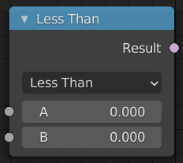# Float Compare¶Float Compare Node.

The Float Compare node takes two inputs and does a math comparison between them.

## Inputs¶

A, B

Standard float value input.

Epsilon

This value is used as a threshold for still considering the two inputs as equal for the Equal and Not Equal operations.

## Properties¶

Mode
Less Than

True if A is smaller than B.

Less than or Equal

True if A is smaller or equal than B.

Greater Than

True if A is bigger than B.

Greater than or Equal

True if A is bigger or equal than B.

Equal

True if A and B are the same.

Not Equal

True if A and B are different.

## Output¶

Result

Standard Boolean output.